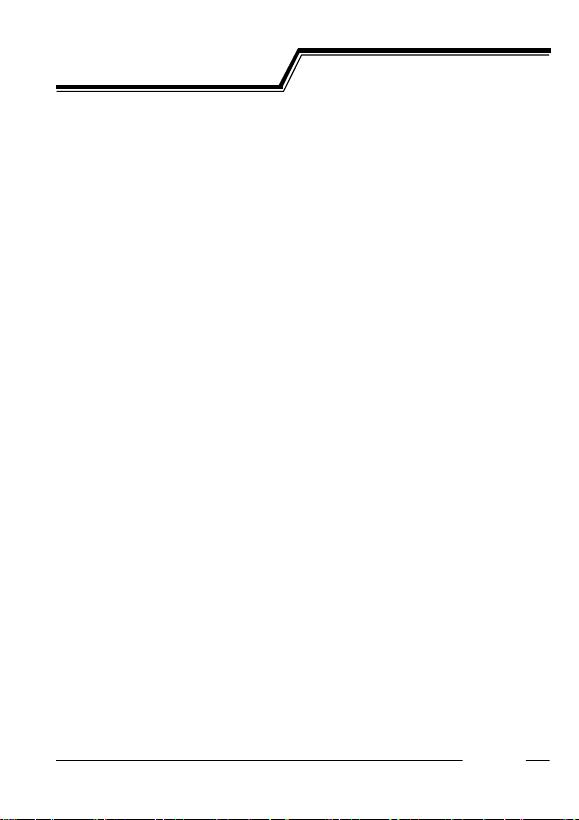PWM/VFM step-down DC/DC Converter
R1224N Series
2001.10.18
12345 PRELIMINARY
12345
Rev. 0.06 - 1 -
OUTLINE
The R1224N Series are CMOS-based PWM step-down DC/DC Converter controllers with low supply current.
Each of these ICs consists of an oscillator, a PWM control circuit, a reference voltage unit, an error amplifier, a
phase compensation circuit, a soft-start circuit, a protection circuit, a PWM/VFM alternative circuit, a chip enable
circuit, resistors for output voltage detect, and input voltage detect circuit. A low ripple, high efficiency step-down
DC/DC converter can be easily composed of this IC with only several external components, or a power-transistor, an
inductor, a diode and capacitors. Output Voltage is fixed or can be adjusted with external resistors (Adjustable types
are without PWM/VFM alternative circuit).
With a PWM/VFM alternative circuit, when the load current is small, the operation is automatically switching into
the VFM oscillator from PWM oscillator. Therefore, the efficiency at small load current is improved. Several types of
the R1224N XXX, which are without a PWM/VFM alternative circuit, are also available.
If the term of maximum duty cycle keeps on a certain time, the embedded protection circuit works. The protection
circuit is Reset-type protection circuit, and it works to restart the operation with soft-start and repeat this operation until
maximum duty cycle condition is released. When the cause of large load current or something else is removed, the
operation is automatically released and returns to normal operation.
Further, built-in UVLO function works when the input voltage is equal or less than UVLO threshold, it makes this IC
be standby and suppresses the consumption current and avoid an unstable operation.
FEATURES
Range of Input Voltage 2.3V18.5V
Built-in Soft-start Function and Protection Function (Reset type protection)
Three options of Oscillator Frequency 180kHz, 300kHz, 500kHz
High Efficiency TYP. 90%
Output Voltage Stepwise Setting with a step of 0.1V in the range of 1.2V to 6.0V as
fixed voltage type. Reference Voltage of Adjustable Type is 1.0V
Standby Current TYP. 0.0µA
High Accuracy Output Voltage ±2.0%
Low Temperature-Drift Coefficient of Output Voltage TYP. ±100ppm/°C
APPLICATIONS
Power source for hand-held communication equipment, cameras, video instruments such as VCRs,
camcorders.
Power source for battery-powered equipment.
Power source for household electrical appliances.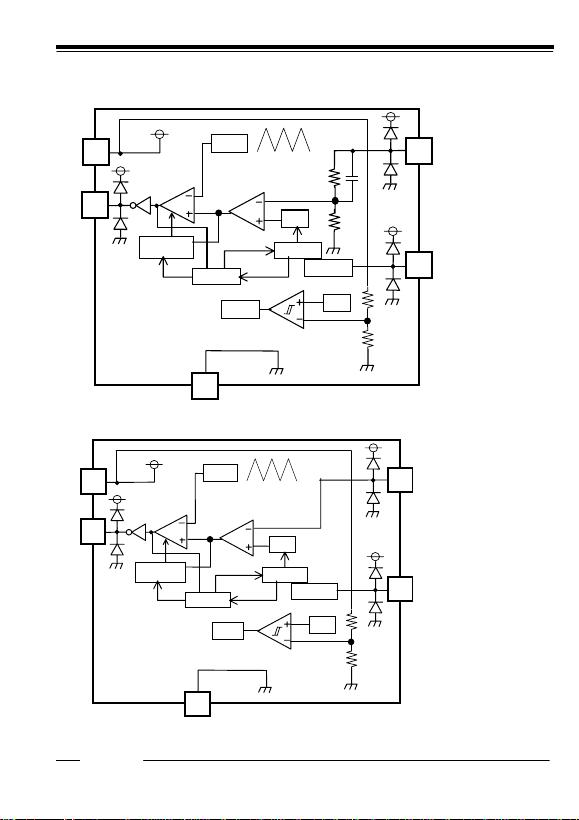12345
Rev. 0.06 - 2 -
BLOCK DIAGRAM
*Fixed Output Voltage Type
OSC
V
IN
EXT
GND
VOUT
Vref
CE
Chip Enable
Soft Start
Protection
PWM/VFM
CONTROL
Vref
Amp
UVLO
OSC
V
IN
EXT
GND
VFB
Vref
CE
Chip Enable
Soft Start
Protection
PWM/VFM
CONTROL
Vref
UVLO
Amp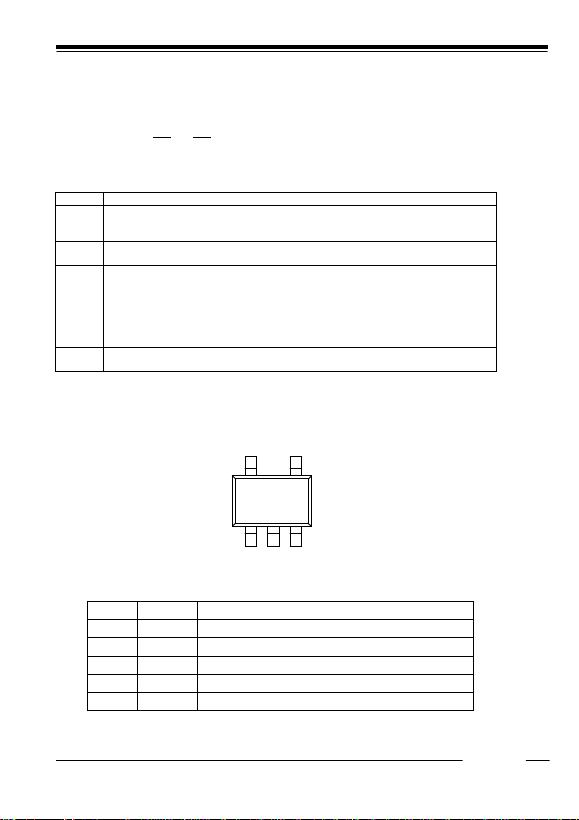12345
Rev. 0.06 - 3 -
SELECTION GUIDE
In the R1224N Series, the output voltage, the oscillator frequency, the optional function, and the taping type for
the ICs can be selected at the users request.
The selection can be made with designating the part number as shown below;
R1224NXX2X-XX
a b c d
Code Contents
a Setting Output Voltage(VOUT):
Stepwise setting with a step of 0.1V in the range of 1.2V to 6.0V is possible.
Adjustable type; a=10 means Reference voltage=1.0V Optional Function is G/H/M.
b Designation of Oscillator Frequency
2 : fixed
c Designation of Optional Function
E : 300kHz, with a PWM/VFM alternative circuit
F : 500kHz, with a PWM/VFM alternative circuit
G : 300kHz, without a PWM/VFM alternative circuit
H : 500kHz, without a PWM/VFM alternative circuit
L : 180kHz, with a PWM/VFM alternative circuit
M : 180kHz, without a PWM/VFM alternative circuit
d Designation of Taping Type; Ex. :TR,TL(refer to Taping Specification)
”TR” is prescribed as a standard.
PIN CONFIGURATION
SOT-23-5
1 2
3
4
V
IN EXT
V
OUT
CE GND (VFB)
(mark side)
5
PIN DESCRIPTION
Pin No. Symbol Description
1 CE Chip Enable Pin (Active with “H”)
2 GND Ground Pin
3V
OUT /(VFB) Pin for Monitoring Output Voltage(Feedback Voltage)
4 EXT External Transistor Drive Pin(CMOS Output)
5V
IN Power Supply Pin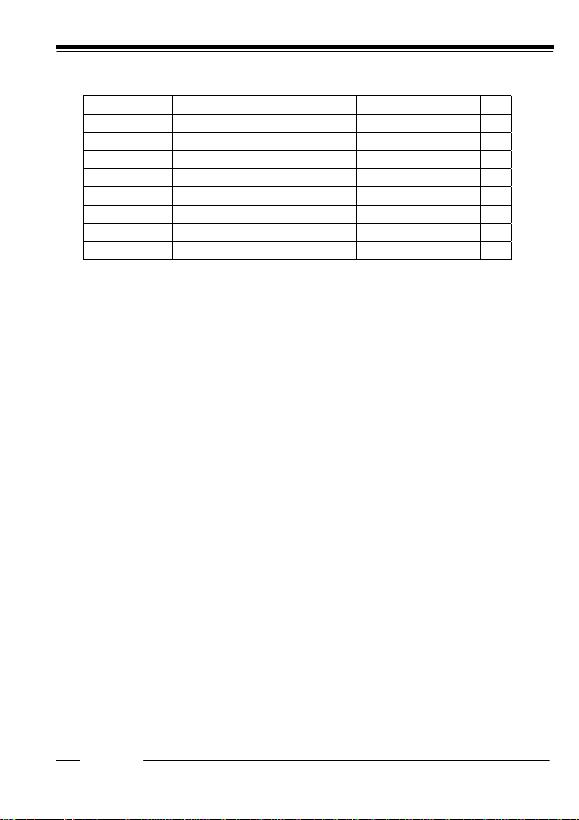12345
Rev. 0.06 - 4 -
ABSOLUTE MAXIMUM RATINGS
(GND=0V)
Symbol Item Rating Unit
VIN V
IN Supply Voltage 20 V
VEXT EXT Pin Output Voltage -0.3VIN+0.3 V
VCE CE Pin Input Voltage -0.3VIN+0.3 V
VOUT/(VFB) V
OUT/VFB Pin Input Voltage -0.3VIN+0.3 V
IEXT EXT Pin Inductor Drive Output Current ±50 mA
PD Power Dissipation 250 mW
Topt Operating Temperature Range -40+85 °C
Tstg Storage Temperature Range -55+125 °C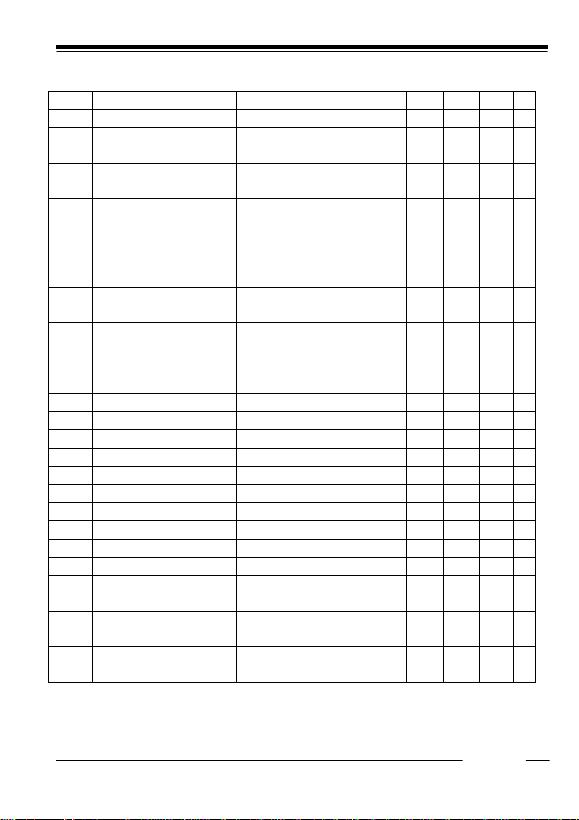12345
Rev. 0.06 - 5 -
ELECTRICAL CHARACTERISTICS
R1224Nxx2X (X=E/F/G/H/L/M) except R1224N102X (Topt=25°C)
Symbol Item Conditions MIN. TYP. MAX. Unit
VIN Operating Input Voltage 2.3 18.5 V
VOUT Step-down Output Voltage VIN=VCE+VSET+1.5V, IOUT=-100mA
When VSET2.0, then VIN=VCE=3.5V
VSET×
0.98
VSET VSET×
1.02
V
VOUT/
T
Step-down Output Voltage
Temperature Coefficient
-40
°C Topt 85°C±100 ppm
/°C
fosc Oscillator Frequency VIN=VCE=VSET+1.5V, IOUT=-100mA
When VSET2.0, then VIN=VCE=3.5V
L/M version
E/G version
F/H version
144
240
400
180
300
500
216
360
600
kHz
fOSC/
T
Oscillator Frequency
Temperature Coefficient
-40°C Topt 85°C±0.2 %
/°C
IDD1 Supply Current1 VIN=VCE=VOUT=18.5V
E/F/L/M version
G version
H version
20
30
40
50
70
90
µA
Istb Standby Current VIN=18.5V, VCE=0V, VOUT=0V 0.0 0.5 µA
IEXTH EXT "H" Output Current VIN=8V,VEXT=7.9V,VOUT=8V,VCE=8V -17 mA
IEXTL EXT "L" Output Current VIN=8V,VEXT=0.1V,VOUT=0V,VCE=8V 30 mA
ICEH CE "H" Input Current VIN=VCE=VOUT=18.5V 0.0 0.5 µA
ICEL CE "L" Input Current VIN= VOUT=18.5V, VCE=0V -0.5 0.0 µA
VCEH CE "H" Input Voltage VIN=8V,VOUT=0V 1.5 V
VCEL CE "L" Input Voltage VIN=8V,VOUT=0V 0.3 V
Maxdty Oscillator Maximum Duty Cycle 100 %
VFMdty VFM Duty Cycle E/F/L version 35 %
VUVLO1 UVLO Voltage VIN=VCE=2.5V to 1.5V, VOUT=0V 1.9 2.0 2.2 V
VUVLO2 UVLO Release Voltage VIN=VCE=1.5V to 2.5V, VOUT=0V VUVLO1
+0.1
2.3 V
Tstart Delay Time by Soft-Start function VIN=VSET+1.5V, IOUT=-10mA
VCE=0V->VSET+1.5V
10 ms
Tprot Delay Time for protection circuit VIN=VCE=VSET+1.5V
VOUT=VSET+1.5V->0V
15 ms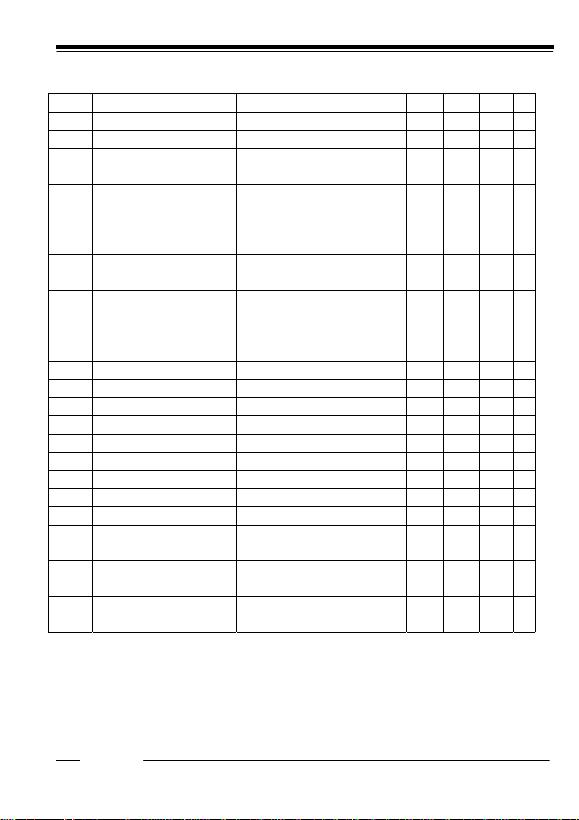12345
Rev. 0.06 - 6 -
R1224N102X (X=G/H/M) (Topt=25°C)
Symbol Item Conditions MIN. TYP. MAX. Unit
VIN Operating Input Voltage 2.3 18.5 V
VFB Feedback Voltage VIN=VCE=3.5V, IFB=-100mA 0.98 1.00 1.02 V
VFB/
T
Feedback Voltage
Temperature Coefficient
-40
°C Topt 85°C±100 ppm
/°C
fosc Oscillator Frequency VIN=VCE=3.5V, IFB=-100mA
M version
G version
H version
144
240
400
180
300
500
216
360
600
kHz
fOSC/
T
Oscillator Frequency
Temperature Coefficient
-40°C Topt 85°C±0.2 %
/°C
IDD1 Supply Current1 VIN=VCE=VFB=18.5V
M version
G version
H version
20
30
40
50
70
90
µA
Istb Standby Current VIN=18.5V, VCE=0V, VFB=0V 0.0 0.5 µA
IEXTH EXT "H" Output Current VIN=8V,VEXT=7.9V,VFB=8V,VCE=8V -17 mA
IEXTL EXT "L" Output Current VIN=8V,VEXT=0.1V,VFB=0V,VCE=8V 30 mA
ICEH CE "H" Input Current VIN=VCE=VFB=18.5V 0.0 0.5 µA
ICEL CE "L" Input Current VIN= VFB=18.5V, VCE=0V -0.5 0.0 µA
VCEH CE "H" Input Voltage VIN=8V,VFB=0V 1.5 V
VCEL CE "L" Input Voltage VIN=8V,VFB=0V 0.3 V
Maxdty Oscillator Maximum Duty Cycle 100 %
VUVLO1 UVLO Voltage VIN=VCE=2.5V to 1.5V, VFB=0V 1.9 2.0 2.2 V
VUVLO2 UVLO Release Voltage VIN=VCE=1.5V to 2.5V, VFB=0V VUVLO1
+0.1
2.3 V
Tstart Delay Time by Soft-Start function VIN=2.5V, IFB=-10mA
VCE=0V->2.5V
10 ms
Tprot Delay Time for protection circuit VIN=VCE=2.5V
VFB=2.5V->0V
15 ms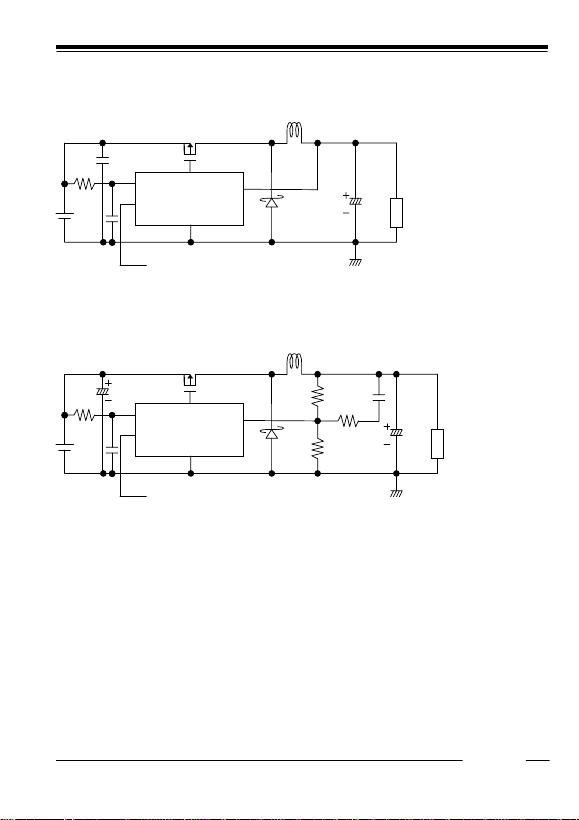12345
Rev. 0.06 - 7 -
TYPICAL APPLICATION AND APPLICATION HINTS
(1) Fixed Output Voltage Type(R1224Nxx2E/F/G/H/L/M except xx=10)
C3
C2
L
C1
R1
SD
VOUT
VIN
CE
CE CONTROL
EXT
GND
PMOS
PMOS: HAT1044M (Hitachi) L: CR105-270MC (Sumida, 27µH)
SD1: RB063L-30 (Rohm) C3: 47µF (Tantalum Type)
C1: 10
µF (Ceramic Type) C2: 0.1µF (Ceramic Type)
R1: 10
(2) Adjustable Output Type (R1224N102G/H/M) Example: Output Voltage=3.2V
C3
C2
L
C1
R1
SD
VFB
VIN
CE
CE CONTROL
EXT
GND
C4
R2
R4
R3
PMOS
PMOS: HAT1044M (Hitachi) L: CR105-270MC (Sumida, 27µH)
SD1: RB063L-30 (Rohm) C3: 47µF (Tantalum Type)
C1: 10µF (Ceramic Type) C2: 0.1µF (Ceramic Type) C4: 2200pF(Ceramic Type)
R1: 10, R2=10k, R3=2.7k, R4=22k
When you use these ICs, consider the following issues;
As shown in the block diagram, a parasitic diode is formed in each terminal, each of these diodes is not formed for
load current, therefore do not use it in such a way. When you control the CE pin by another power supply, do not make
its "H" level more than the voltage level of VIN pin.
Set external components as close as possible to the IC and minimize the connection between the components and
the IC. In particular, a capacitor should be connected to VOUT pin with the minimum connection. And make sufficient
grounding and reinforce supplying. A large switching current could flow through the connection of power supply, an
inductor and the connection of VOUT. If the impedance of the connection of power supply is high, the voltage level of
power supply of the IC fluctuates with the switching current. This may cause unstable operation of the IC.
Protection circuit may work if the maximum duty cycle continue for the time defined in the electrical characteristics.
Once after stopping the output voltage, output will restart with soft-start operation. If the difference between input
voltage and output voltage is small, the protection circuit may work.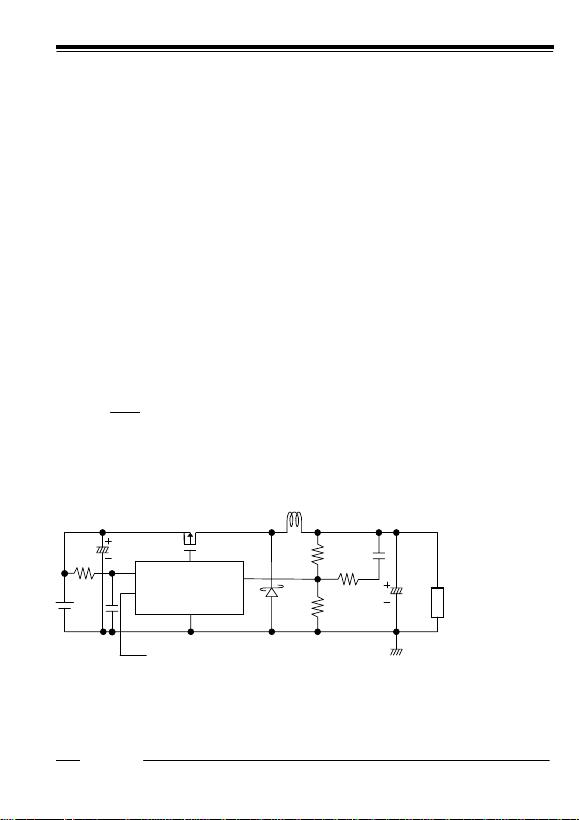12345
Rev. 0.06 - 8 -
Use capacitors with a capacity of 22µF or more for VOUT pin, and with good high frequency characteristics such as
tantalum capacitors. We recommend you to use output capacitors with an allowable voltage at least twice as much as
setting output voltage. This is because there may be a case where a spike-shaped high voltage is generated by an
inductor when an external transistor is on and off.
Choose an inductor that has sufficiently small D.C. resistance and large allowable current and is hard to reach
magnetic saturation. And if the value of inductance of an inductor is extremely small, the ILX may exceed the absolute
Use an inductor with appropriate inductance.
Use a diode of a Schottky type with high switching speed, and also pay attention to its current capacity.
Do not use this IC under the condition with VIN voltage at equal or less than minimum operating voltage.
The performance of power source circuits using these ICs extremely depends upon the peripheral circuits.
Pay attention in the selection of the peripheral circuits. In particular, design the peripheral circuits in a way that the
values such as voltage, current, and power of each component, PCB patterns and the IC do not exceed their
respected rated values.
As for Adjustable Output type, feedback pin (VFB) voltage is controlled to maintain 1.0V.
Output Voltage, VOUT is as following equation:
VOUT: R2+R4=VFB: R2
V
OUT=VFB×(R2+R4)/R2
Thus, with changing the value of R2 and R4, output voltage can be set in the specified range.
In the DC/DC converter, with the load current and external components such as L and C, phase might be behind 180
degree. In this case, the phase margin of the system will be less and stability will be worse. To prevent this, phase
margin should be secured with proceeding the phase. A pole is formed with external components L and C3.
Fpole 1/2π√L×C3
A zero (signal back to zero) is formed with R4 and C4.
Fzero1/(2π×R4×C4)
For example, if L=27µH, C3=47µF, the cut off frequency of the pole is approximately 4.5kHz.
To make the cut off frequency of the pole as much as 4.5kHz, set R4=33k and C4=1000pF.
If VOUT is set at 2.5V, R2=22k is appropriate.
R3 prevents feedback of the noise to VFB pin, about 2.7k is appropriate value.
C3
C2
L
C1
R1
SD
VFB
VIN
CE
CE CONTROL
EXT
GND
C4
R2
R4
R3
PMOS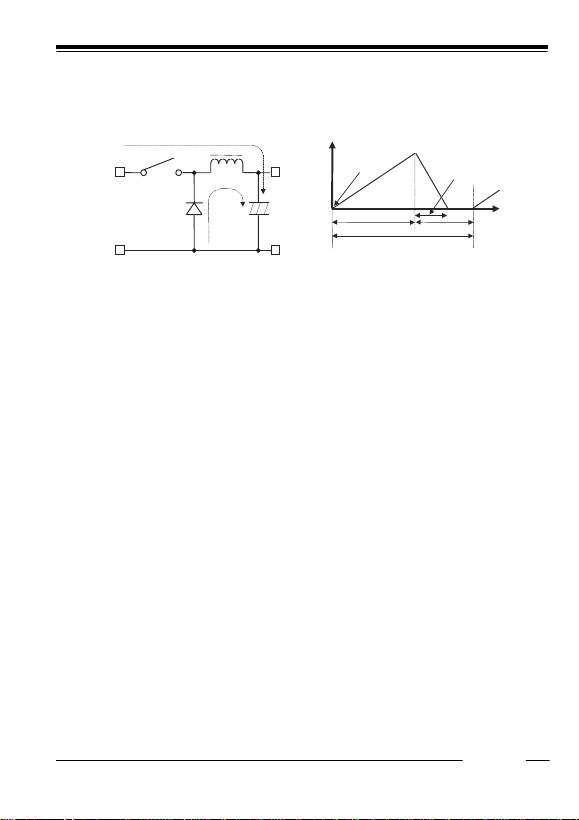12345
Rev. 0.06 - 9 -
OPERATION of step-down DC/DC converter and Output Current
The step-down DC/DC converter charges energy in the inductor when Lx transistor is ON, and discharges the energy
from the inductor when Lx transistor is OFF and controls with less energy loss, so that a lower output voltage than the
input voltage is obtained. The operation will be explained with reference to the following diagrams:
<Basic Circuits> <Current through L>
Step 1: Lx Tr. turns on and current IL (=i1) flows, and energy is charged into CL. At this moment, IL increases from
ILmin. (=0) to reach ILmax. in proportion to the on-time period(ton) of LX Tr.
Step 2: When Lx Tr. turns off, Schottky diode (SD) turns on in order that L maintains IL at ILmax, and current IL (=i2)
flows.
Step 3: IL decreases gradually and reaches ILmin. after a time period of topen, and SD turns off, provided that in the
continuous mode, next cycle starts before IL becomes to 0 because toff time is not enough. In this case, IL value is
from this ILmin (>0).
In the case of PWM control system, the output voltage is maintained by controlling the on-time period (ton), with the
oscillator frequency (fosc) being maintained constant.
Discontinuous Conduction Mode and Continuous Conduction Mode
The maximum value (ILmax) and the minimum value (ILmin) current which flow through the inductor is the same as
those when Lx Tr. is ON and when it is OFF.
The difference between ILmax and ILmin, which is represented by I;
I = ILmax – ILmin = VOUT × topen / L = (VIN-VOUT)×ton/L⋅⋅⋅Equation 1
wherein, T=1/fosc=ton+toff
duty (%)=ton/T
×100=ton× fosc × 100
topen toff
In Equation 1, VOUT×topen/L and (VIN-VOUT)×ton/L are respectively shown the change of the current at ON, and the
change of the current at OFF.
When the output current (IOUT) is relatively small, topen < toff as illustrated in the above diagram. In this case, the
energy is charged in the inductor during the time period of ton and is discharged in its entirely during the time period of
toff, therefore ILmin becomes to zero (ILmin=0). When Iout is gradually increased, eventually, topen becomes to toff
(topen=toff), and when IOUT is further increased, ILmin becomes larger than zero (ILmin>0). The former mode is
referred to as the discontinuous mode and the latter mode is referred to as continuous mode.
In the continuous mode, when Equation 1 is solved for ton and assumed that the solution is tonc,
tonc=T
×VOUT/VIN⋅⋅⋅ Equation 2
When ton<tonc, the mode is the discontinuous mode, and when ton=tonc, the mode is the continuous mode.
SD
L
CL
VOUT
IOUT
VIN Lx Tr
i1
i2
T=1/fosc
toffton
ILmax
ILmin to
p
en12345
Rev. 0.06 - 10 -
OUTPUT CURRENT AND SELECTION OF EXTERNAL COMPONENTS
When Lx Tr. is ON:
(Wherein, Ripple Current P-P value is described as IRP, ON resistance of LX Tr. is described as Rp the direct current
of the inductor is described as RL.)
V
IN=VOUT+(Rp+RL)×IOUT+L×IRP/ton ⋅⋅⋅Equation 3
When Lx Tr. is OFF:
L
×IRP/toff = VF+VOUT+RL×IOUT ⋅⋅⋅Equation 4
Put Equation 4 to Equation 3 and solve for ON duty, ton/(toff+ton)=DON,
D
ON=(VOUT+VF+RL×IOUT)/(VIN+VF-Rp×IOUT)⋅⋅⋅Equation 5
Ripple Current is as follows;
I
RP=(VIN-VOUT-Rp×IOUT-RL×IOUT)×DON/f/L Equation 6
Wherein, peak current that flows through L, Lx Tr., and SD is as follows;
ILmax=I
OUT+IRP/2Equation 7
Consider ILmax, condition of input and output and select external components.
The above explanation is directed to the calculation in an ideal case in continuous mode.
External Components
1. Inductor
Select an inductor that peak current does not exceed ILmax. If larger current than allowable current flows, magnetic
saturation occurs and make transform efficiency worse.
When the load current is definite, the smaller value of L, the larger the ripple current.
Provided that the allowable current is large in that case and DC current is small, therefore, for large output current,
efficiency is better than using an inductor with a large value of L and vice versa.
2. Diode
Use a diode with low VF (Schottky type is recommended.) and high switching speed.
Reverse voltage rating should be more than VIN and current rating should be equal or more than ILmax.
3. Capacitors
As for CIN, use a capacitor with low ESR (Equivalent Series Resistance) and a capacity of at least 10µF for stable
operation.
COUT can reduce ripple of Output Voltage, therefore 47µF or more value of tantalum type capacitor is
recommended.
4. Lx Transistor
Pch Power MOSFET is required for this IC.
Its breakdown voltage between gate and source should be a few V higher than Input Voltage.
In the case of Input Voltage is low, to turn on MOSFET completely, to use a MOSFET with low threshold voltage is
effective.
If a large load current is necessary for your application and important, choose a MOSFET with low ON resistance
for good efficiency.
If a small load current is mainly necessary for your application, choose a MOSFET with low gate capacity for good
efficiency.
Maximum continuous drain current of MOSFET should be larger than peak current, ILmax.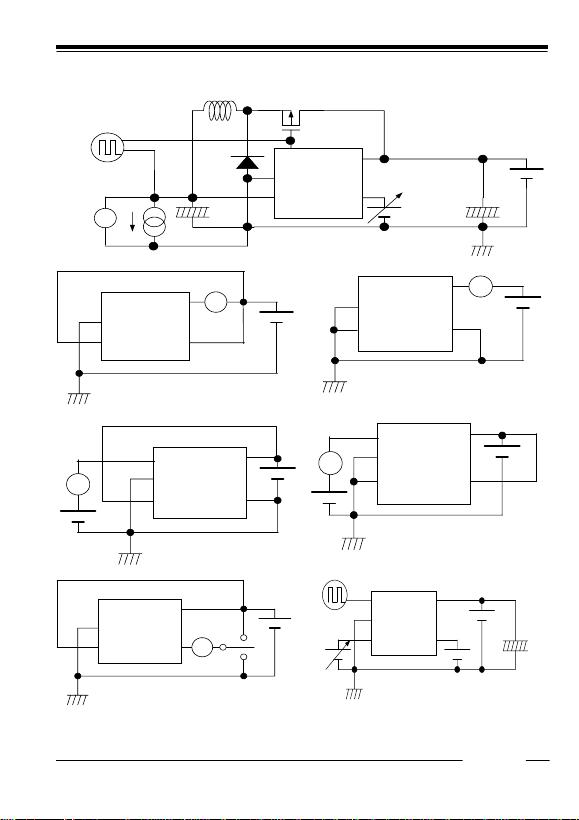12345
Rev. 0.06 - 11 -
TEST CIRCUITS
A) Output Voltage, Oscillator Frequency, CE”H” Input Voltage, CE”L” Input Voltage, Soft-start time
EXT
GND
VOUT
(VFB)
VIN
CE
PMOS
L1
+
-
C1 +
-
C2
D1
Oscilloscope
V
B) Supply Current1 C) Standby Current
EXT
GND
VOUT
(VFB)
VIN
CE
A
EXT
GND
VOUT
(VFB)
VIN
CE
A
D) EXT “H” Output Current E) EXT “L” Output Current
EXT
GND
VOUT
(VFB)
VIN
CE
A
EXT
GND
VOUT
(VFB)
VIN
CE
A
F) CE “H” Input Current, CE “L” Input Current G) Output Delay Time for Protection Circuit
EXT
GND
VOUT
(VFB)
VIN
CE A
Oscilloscope
EXT
GND
VOUT
(VFB)
VIN
CE
+
-
C2
PMOS: HAT1044M (Hitachi) L: CD104-270MC (Sumida, 27µH)
SD1: RB491D (Rohm)
C1: 47µF (Tantalum Type) C2: 47µF (Tantalum Type)